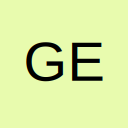# Multiple Lookup Function in one Formula

• Quite simply I'm having a lot of trouble attempting to resolve this issue. - However I think my issue is that I MAY be using the incorrect function.

I'm attempting to use a LOOKUP function more than once in a single cell.

Here's the forumla that currently works:

=IF(G21="N/A",0,IF(G21="Perf",LOOKUP(H21,R7:U7,IF(D7<=4572,R8:U8,IF(D7<=7620,R9:U9,IF(D7<=10668,R10:U10,R11:U11))))*(((D7*D8)+((D7*D9)*2)+(D8*D9))/1000000)))

Ie. If "N/A" is Selected, then returns a value of zero. (Works). If "Perf" is selected then the cell returns an answer based on a value using a LOOKUP function (Also Works!)

However, if I attempt to add another LOOKUP function in to that cell such as:

IF(G21="N/A",0,IF(G21="Perf",LOOKUP(H21,R7:U7,IF(D7<=4572,R8:U8,IF(D7<=7620,R9:U9,IF(D7<=10668,R10:U10,R11:U11))))*(((D7*D8)+((D7*D9)*2)+(D8*D9))/1000000))),IF(G21="Zintec",(LOOKUP(H21,R13:U13,IF(D7<=4572,R14:U14,IF(D7<=7620,R15:U15,IF(D7<=10668,R16:U16,R17:U17))))*(((D7*D8)+((D7*D9)*2)+(D8*D9))/1000000)))

All I seem to get is #VALUE! no matter what I choose in the drop down cell validation cell.

Is it not possible to use the LOOKUP function more than once in a cell?

• Re: Multiple Lookup Function in one Formula.

I'd guess parentheses in the wrong place:

=IF(G21="N/A",0,IF(G21="Perf",LOOKUP(H21,R7:U7,IF(D7<=4572,R8:U8,IF(D7<=7620,R9:U9,IF(D7<=10668,R10:U10,R11:U11))))*(((D7*D8)+((D7*D9)*2)+(D8*D9))/1000000),IF(G21="Zintec",(LOOKUP(H21,R13:U13,IF(D7<=4572,R14:U14,IF(D7<=7620,R15:U15,IF(D7<=10668,R16:U16,R17:U17))))*(((D7*D8)+((D7*D9)*2)+(D8*D9))/1000000)))))

Cheers, Glenn.

Beauty is in the eye of the beer-holder.

• Re: Multiple Lookup Function in one Formula.

Quote from GlennUK;569610

I'd guess parentheses in the wrong place:

=IF(G21="N/A",0,IF(G21="Perf",LOOKUP(H21,R7:U7,IF(D7<=4572,R8:U8,IF(D7<=7620,R9:U9,IF(D7<=10668,R10:U10,R11:U11))))*(((D7*D8)+((D7*D9)*2)+(D8*D9))/1000000),IF(G21="Zintec",(LOOKUP(H21,R13:U13,IF(D7<=4572,R14:U14,IF(D7<=7620,R15:U15,IF(D7<=10668,R16:U16,R17:U17))))*(((D7*D8)+((D7*D9)*2)+(D8*D9))/1000000)))))

You're actually absolutely right and that works fine, although I can't work out why I can't add another LOOKUP command within that cell (I've only two more to do!) I didn't ask this first time around because I wanted to resolve this issue myself - so I assumed with one example I could figure out the rest, but evidently not.

So, now I've got:

IF(G21="N/A",0,IF(G21="Perf",LOOKUP(H21,R7:U7,IF(D7<=4572,R8:U8,IF(D7<=7620,R9:U9,IF(D7<=10668,R10:U10,R11:U11))))*(((D7*D8)+((D7*D9)*2)+(D8*D9))/1000000),IF(G21="Zintec",(LOOKUP(H21,R13:U13,IF(D7<=4572,R14:U14,IF(D7<=7620,R15:U15,IF(D7<=10668,R16:U16,R17:U17))))*(((D7*D8)+((D7*D9)*2)+(D8*D9))/1000000),IF(G21=316,(LOOKUP(H21,R19:U19,IF(D7<=4572,R20:U20,IF(D7<=7620,R21:U21,IF(D7<=10668,R22:U22,R23:U23))))*(((D7*D8)+((D7*D9)*2)+(D8*D9))/1000000)))))))

"The Formula Entered contains an error".

Really? Even though I copied and pasted it? Which leads me back to my original question thought process. - Is there a limit to the amount of LOOKUP's you can have in one cell?

And by the way, thank you for your quick response.

• Re: Multiple Lookup Function in one Formula

No, but the formula can't exceed 8192 characters

• Re: Multiple Lookup Function in one Formula

Hi GeforceXP,

Do not try to put all logic in formulas.

Create master table, from the table you can use only by two forrmulas you can able to do this formula.
-----------------------------------------------------
A B C
Perf 100 200 300
Zintec 101 201 301
-----------------------------------------------------
A 2
B 3
C 4
-------------
vlookup(perf,range,vlookup(A,range,2,0),0)

Use name ranges if required in formulas as indirect.

Regards
Suresh
Mumbai

• Re: Multiple Lookup Function in one Formula

...yes, your formula contains an error ... I've only got a few seconds today to look at it, and straight away I can say that you don't even have matching parenthses. Will look again on Monday.

Cheers, Glenn.

Beauty is in the eye of the beer-holder.

## Participate now!

Don’t have an account yet? Register yourself now and be a part of our community!